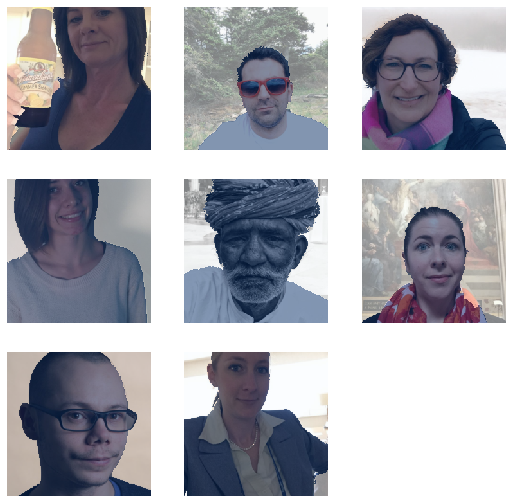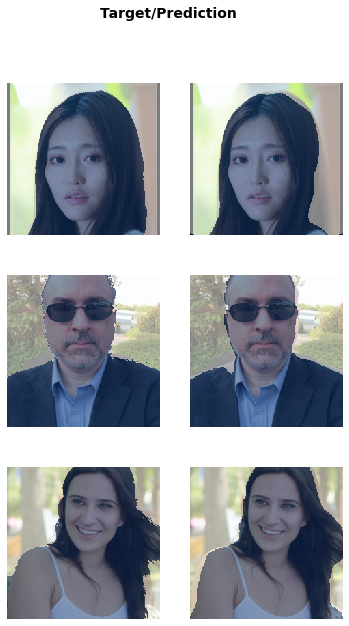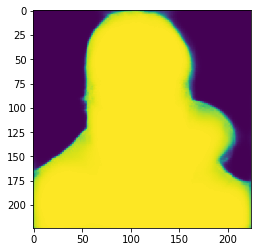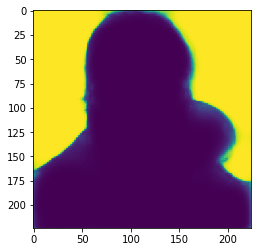This article is also a Jupyter Notebook available to be run from the top down. There will be code snippets that you can then run in any environment.

Below are the versions of fastai, fastcore, and wwf currently running at the time of writing this:

• fastai: 2.1.10
• fastcore: 1.3.13
• wwf: 0.0.7

file = "https://drive.google.com/uc?id=18xM3jU2dSp1DiDqEM6PVXattNMZvsX4z"

!gdown {file}

Downloading...
To: /content/Portrait.zip
107MB [00:02, 44.4MB/s]


We'll unzip the data

from zipfile import ZipFile

with ZipFile('Portrait.zip', 'r') as zip_ref:
zip_ref.extractall('')

from fastai.vision.all import *


And grab our ground truth labels and files

path = Path('')

lbl_names = get_image_files(path/'GT_png')
fnames = get_image_files(path/'images_data_crop')

img_fn = fnames; img_fn

Path('images_data_crop/00970.jpg')
lbl_names

Path('GT_png/02621_mask.png')
fn = '00013.jpg'

im = PILImage.create(f'images_data_crop/{fn}')

msk = PILMask.create(f'GT_png/00013_mask.png')


Now, our mask isn't set up how fastai expects, in which the mask points are not all in a row. We need to change this:

len(np.unique(msk))

2
np.unique(msk)

array([  0, 255], dtype=uint8)

We'll do this through an n_codes function. What this will do is run through our masks and build a set based on the unique values present in our masks. From there we will build a dictionary that will replace our points once we load in the image

def n_codes(fnames, is_partial=True):
"Gather the codes from a list of fnames"
vals = set()
if is_partial:
random.shuffle(fnames)
fnames = fnames[:10]
for fname in fnames:
for val in np.unique(msk):
if val not in vals:
vals = list(vals)
p2c = dict()
for i,val in enumerate(vals):
p2c[i] = vals[i]
return p2c

p2c = n_codes(lbl_names)


So p2c in this case is anywhere that is 255 in our mask should be replaced to one

p2c

{0: 0, 1: 255}

So now let's build a get_msk function that will modify our mask we get based on this dictionary and override those values

def get_msk(fn, p2c):
"Grab a mask from a filename and adjust the pixels based on pix2class"
mx = np.max(msk)
for i, val in enumerate(p2c):
msk[msk==p2c[i]] = val

codes = ['Background', 'Face']


Now we can build a get_y and a DataBlock!

get_y = lambda o: get_msk(o, p2c)

binary = DataBlock(blocks=(ImageBlock, MaskBlock(codes)),
get_items=get_image_files,
splitter=RandomSplitter(),
get_y=get_y,
item_tfms=Resize(224),
batch_tfms=[Normalize.from_stats(*imagenet_stats)])

dls = binary.dataloaders(path/'images_data_crop', bs=8)


We can look at how our masks look by adjusting the colormap and the vmin and max

dls.show_batch(cmap='Blues', vmin=0, vmax=1)And now we can train!

learn = unet_learner(dls, resnet34)

Downloading: "https://download.pytorch.org/models/resnet34-333f7ec4.pth" to /root/.cache/torch/checkpoints/resnet34-333f7ec4.pth


learn.fit(1)

epoch train_loss valid_loss time
0 0.287433 0.168146 00:43

And we're good :)

learn.show_results(cmap='Blues', vmin=0, vmax=1)If we want to examine it further we can do:

preds = learn.get_preds()

preds.shape

torch.Size([2, 224, 224])
p = preds

plt.imshow(p)

<matplotlib.image.AxesImage at 0x7f942a6599e8>plt.imshow(p)

<matplotlib.image.AxesImage at 0x7f942a5d8240>